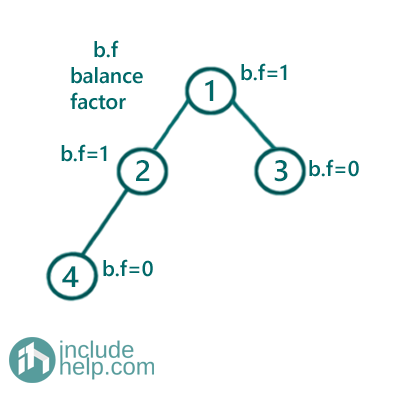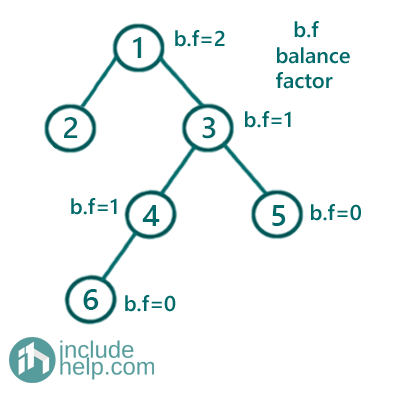# Check If a Given Tree is Height-balanced or Not

In this article, we are going to discuss what a height-balanced tree is and how to find whether a tree is height-balanced or not?
Submitted by Radib Kar, on August 08, 2020

## What is a height-balanced tree?

A tree is said to be height-balanced if the difference b/w its left subtree height and right subtree height is at most 1.

So, from the below example you can get an idea of which one is a height-balanced tree and which one is not. For checking height balancing, we use a term called balance factor. Each node has a balance factor which is different b/w height of its subtrees.

Balance factor: abs(left subtree height – right subtree height)

Now,

For a tree to be a height-balanced tree, the balance factor of all node in the tree needs to be <=1

For the below example,

We have shown the trees with balance a factor labeled against each node.

Example 1:In the above tree, we can see that for any node the balance factor is at most 1. Thus the above tree is height-balanced.

Example 2:In the above tree, we can see that for the root node the balance factor is 2 which violates the property of the height-balanced tree. Thus the above tree is not height-balanced

So how can we check whether a tree is height-balanced or not?

So, the idea is to check the balance factor for the root. If the balance factor for the root is less than or equal to 1 then we should check whether its subtrees are both height-balanced or not. If all these conditions satisfy then the tree is height-balanced otherwise not. So, In simple recursive definition,

```Bool isBalanced(root){
If left subtree height and right subtree height has
an absolute difference more than 1 then return false
Return isBalanced(root->left) &&  isBalanced(root->right)
}
```

C++ Implementation:

```#include <bits/stdc++.h>
using namespace std;

// tree node is defined
class TreeNode {
public:
int val;
TreeNode* left;
TreeNode* right;
TreeNode(int data)
{
val = data;
left = NULL;
right = NULL;
}
};

int height(TreeNode* root)
{
if (!root)
return 0;
return 1 + max(height(root->left), height(root->right));
}

bool isBalanced(TreeNode* root)
{
if (!root)
return true;

//balancefactor=diff b/w left subtree height
//and right subtree height
int balancefactor = abs(height(root->left) - height(root->right));

//if balance factor of current node >1
//then it can't be height-balanced
if (balancefactor > 1)
return false;
//else if both subtrees are height
//balanced then it's height-balanced too
return isBalanced(root->left) && isBalanced(root->right);
}

int main()
{
//building the tree like example 1
TreeNode* root1 = new TreeNode(1);

root1->left = new TreeNode(2);
root1->right = new TreeNode(3);
root1->left->left = new TreeNode(4);

if (isBalanced(root1))
cout << "Tree in example 1 is height-balanced\n";
else
cout << "Tree in example 1 is not height-balanced\n";

//building the tree-like example 2
TreeNode* root2 = new TreeNode(1);

root2->left = new TreeNode(2);
root2->right = new TreeNode(3);
root2->right->left = new TreeNode(4);
root2->right->left = new TreeNode(5);
root2->right->left->left = new TreeNode(6);

if (isBalanced(root2))
cout << "Tree in example 2 is height-balanced\n";
else
cout << "Tree in example 2 is not height-balanced\n";

return 0;
}
```

Output:

```Tree in example 1 is height-balanced
Tree in example 2 is not height-balanced
```

The time complexity of the above implementation is O(n^2) as it will keep computing height for each recursive call. That's what we can do instead of computing the height every time, we can store the height of each node in one iteration which will take O(n). And then we will check recursively without computing the height in each recursive call, rather can find height from the loop up table in which we stored.

Below is the implementation where we have to create a hash table to store the height of each node so that height can be retrieved in O(1) time from the hash table. Below is the efficient implementation which solves in O(2n) time.

C++ Implementation:

```#include <bits/stdc++.h>
using namespace std;

// tree node is defined
class TreeNode {
public:
int val;
TreeNode* left;
TreeNode* right;
TreeNode(int data)
{
val = data;
left = NULL;
right = NULL;
}
};

int height(TreeNode* root, unordered_map<TreeNode*, int>& mymap)
{
//base case
if (!root)
return 0;

//if height already calculated for the node return the height
if (mymap.find(root) != mymap.end())
return mymap[root];

//calculate height
int h = 1 + max(height(root->left, mymap), height(root->right, mymap));
//store in the hash table
mymap[root] = h;
return h;
}

bool isBalancedRecur(TreeNode* root, unordered_map<TreeNode*, int>& mymap)
{
if (!root)
return true;

//balancefactor=diff b/w left subtree height
//and right subtree height
int balancefactor = abs(mymap[root->left] - mymap[root->right]);

//if balance factor of current node >1
//then it can't be height-balanced
if (balancefactor > 1)
return false;
//else if both subtrees are height balanced
//then it's height-balanced too
return isBalancedRecur(root->left, mymap) && isBalancedRecur(root->right, mymap);
}

bool isBalanced(TreeNode* root)
{
unordered_map<TreeNode*, int> mymap;
if (!root)
return true;
height(root, mymap);
return isBalancedRecur(root, mymap);
}

int main()
{
//building the tree like example 1
TreeNode* root1 = new TreeNode(1);

root1->left = new TreeNode(2);
root1->right = new TreeNode(3);
root1->left->left = new TreeNode(4);

if (isBalanced(root1))
cout << "Tree in example 1 is height-balanced\n";
else
cout << "Tree in example 1 is not height-balanced\n";

//building the tree-like example 2
TreeNode* root2 = new TreeNode(1);

root2->left = new TreeNode(2);
root2->right = new TreeNode(3);
root2->right->left = new TreeNode(4);
root2->right->left = new TreeNode(5);
root2->right->left->left = new TreeNode(6);

if (isBalanced(root2))
cout << "Tree in example 2 is height-balanced\n";
else
cout << "Tree in example 2 is not height-balanced\n";

return 0;
}
```

Output:

```Tree in example 1 is height-balanced
Tree in example 2 is not height-balanced
```

What's New

Top Interview Coding Problems/Challenges!

Languages: » C » C++ » C++ STL » Java » Data Structure » C#.Net » Android » Kotlin » SQL
Web Technologies: » PHP » Python » JavaScript » CSS » Ajax » Node.js » Web programming/HTML
Solved programs: » C » C++ » DS » Java » C#
Aptitude que. & ans.: » C » C++ » Java » DBMS
Interview que. & ans.: » C » Embedded C » Java » SEO » HR
CS Subjects: » CS Basics » O.S. » Networks » DBMS » Embedded Systems » Cloud Computing
» Machine learning » CS Organizations » Linux » DOS
More: » Articles » Puzzles » News/Updates